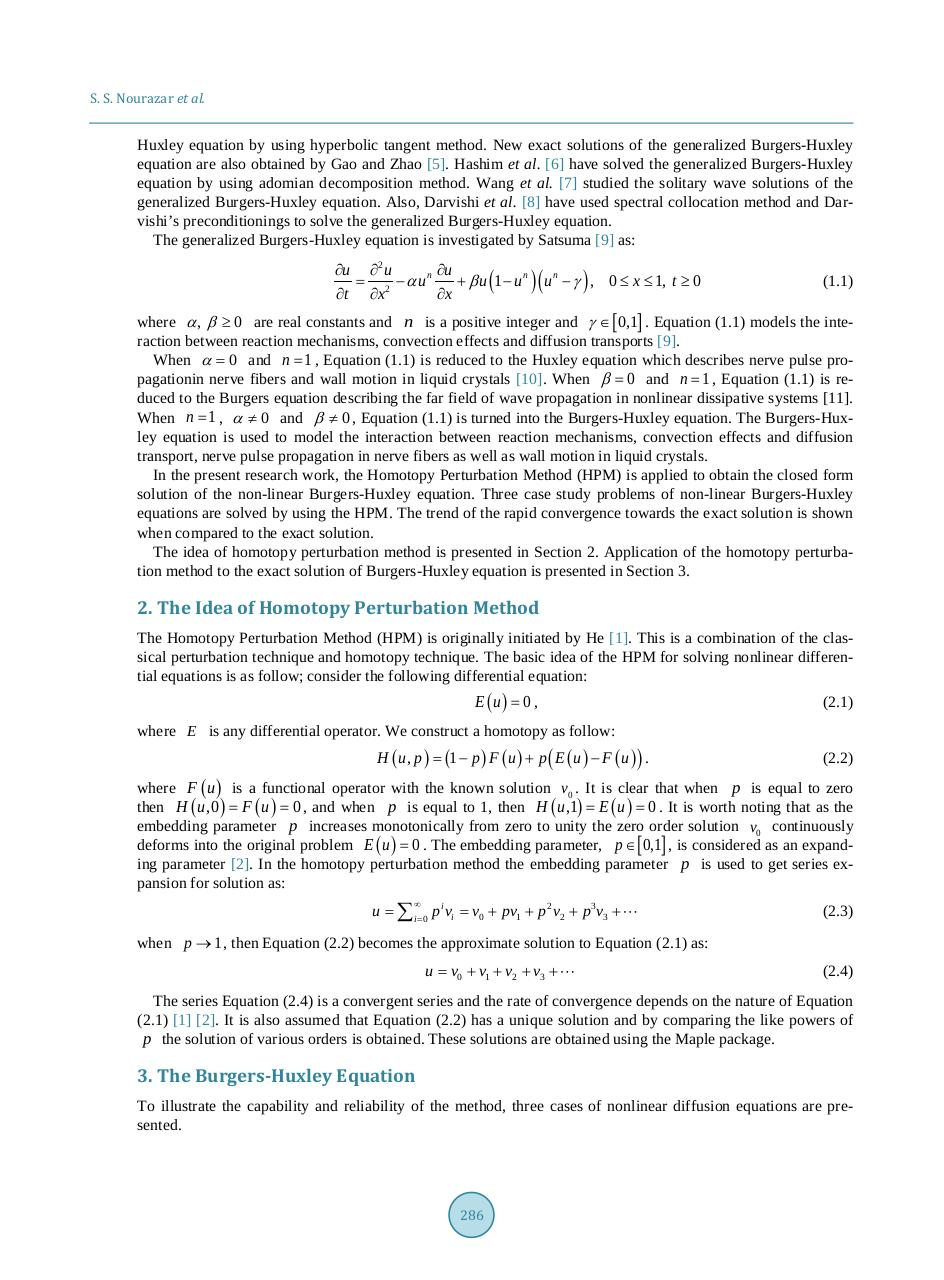# 285 294.pdfPage 1 2 3 4 5 6 7 8 9 10

#### Text preview

S. S. Nourazar et al.

Huxley equation by using hyperbolic tangent method. New exact solutions of the generalized Burgers-Huxley
equation are also obtained by Gao and Zhao . Hashim et al.  have solved the generalized Burgers-Huxley
equation by using adomian decomposition method. Wang et al.  studied the solitary wave solutions of the
generalized Burgers-Huxley equation. Also, Darvishi et al.  have used spectral collocation method and Darvishi’s preconditionings to solve the generalized Burgers-Huxley equation.
The generalized Burgers-Huxley equation is investigated by Satsuma  as:

∂u ∂ 2 u
∂u
=
− αun
+ β u 1 − u n u n − γ , 0 ≤ x ≤ 1, t ≥ 0
2
∂t ∂x
∂x

(

)(

)

(1.1)

where α , β ≥ 0 are real constants and n is a positive integer and γ ∈ [ 0,1] . Equation (1.1) models the interaction between reaction mechanisms, convection effects and diffusion transports .
When α = 0 and n = 1 , Equation (1.1) is reduced to the Huxley equation which describes nerve pulse propagationin nerve fibers and wall motion in liquid crystals . When β = 0 and n = 1 , Equation (1.1) is reduced to the Burgers equation describing the far field of wave propagation in nonlinear dissipative systems .
When n = 1 , α ≠ 0 and β ≠ 0 , Equation (1.1) is turned into the Burgers-Huxley equation. The Burgers-Huxley equation is used to model the interaction between reaction mechanisms, convection effects and diffusion
transport, nerve pulse propagation in nerve fibers as well as wall motion in liquid crystals.
In the present research work, the Homotopy Perturbation Method (HPM) is applied to obtain the closed form
solution of the non-linear Burgers-Huxley equation. Three case study problems of non-linear Burgers-Huxley
equations are solved by using the HPM. The trend of the rapid convergence towards the exact solution is shown
when compared to the exact solution.
The idea of homotopy perturbation method is presented in Section 2. Application of the homotopy perturbation method to the exact solution of Burgers-Huxley equation is presented in Section 3.

2. The Idea of Homotopy Perturbation Method
The Homotopy Perturbation Method (HPM) is originally initiated by He . This is a combination of the classical perturbation technique and homotopy technique. The basic idea of the HPM for solving nonlinear differential equations is as follow; consider the following differential equation:

E (u ) = 0 ,

(2.1)

where E is any differential operator. We construct a homotopy as follow:

H ( u, p ) =
(1 − p ) F ( u ) + p ( E ( u ) − F ( u ) ) .

(2.2)

where F ( u ) is a functional operator with the known solution v0 . It is clear that when p is equal to zero
u,1) E=
u , 0 ) F=
then H (=
( u ) 0 , and when p is equal to 1, then H (=
( u ) 0 . It is worth noting that as the
embedding parameter p increases monotonically from zero to unity the zero order solution v0 continuously
deforms into the original problem E ( u ) = 0 . The embedding parameter, p ∈ [ 0,1] , is considered as an expanding parameter . In the homotopy perturbation method the embedding parameter p is used to get series expansion for solution as:
u =∑ i = 0 p i vi =v0 + pv1 + p 2 v2 + p 3 v3 + 

(2.3)

when p → 1 , then Equation (2.2) becomes the approximate solution to Equation (2.1) as:

u = v0 + v1 + v2 + v3 +

(2.4)

The series Equation (2.4) is a convergent series and the rate of convergence depends on the nature of Equation
(2.1)  . It is also assumed that Equation (2.2) has a unique solution and by comparing the like powers of
p the solution of various orders is obtained. These solutions are obtained using the Maple package.

3. The Burgers-Huxley Equation
To illustrate the capability and reliability of the method, three cases of nonlinear diffusion equations are presented.

286